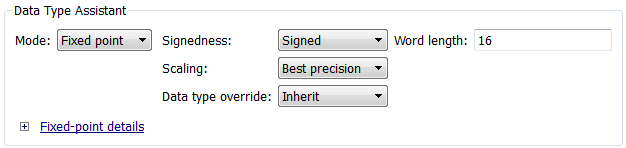Documentation

Fixed-Point Numbers in Simulink

Constant Scaling for Best Precision

The following fixed-point Simulink® blocks provide a mode for scaling parameters whose values are constant vectors or matrices:

This scaling mode is based on binary-point-only scaling. Using this mode, you can scale a constant vector or matrix such that a common binary point is found based on the best precision for the largest value in the vector or matrix.

Constant scaling for best precision is available only for fixed-point data types with unspecified scaling. All other fixed-point data types use their specified scaling. You can use the Data Type Assistant (see Specify Data Types Using Data Type Assistant (Simulink)) on a block dialog box to enable the best precision scaling mode.

1. On a block dialog box, click the button.

The Data Type Assistant appears.

2. In the Data Type Assistant, and from the Mode list, select Fixed point.

The Data Type Assistant displays additional options associated with fixed-point data types.

3. From the Scaling list, select Best precision.To understand how you might use this scaling mode, consider a 3-by-3 matrix of doubles, M, defined as

3.3333e-003  3.3333e-004  3.3333e-005
3.3333e-002  3.3333e-003  3.3333e-004
3.3333e-001  3.3333e-002  3.3333e-003

Now suppose you specify M as the value of the Gain parameter for a Gain block. The results of specifying your own scaling versus using the constant scaling mode are described here:

• Specified Scaling

Suppose the matrix elements are converted to a signed, 10-bit generalized fixed-point data type with binary-point-only scaling of 2-7 (that is, the binary point is located seven places to the left of the right most bit). With this data format, M becomes

0            0            0
3.1250e-002  0            0
3.3594e-001  3.1250e-002  0

Note that many of the matrix elements are zero, and for the nonzero entries, the scaled values differ from the original values. This is because a double is converted to a binary word of fixed size and limited precision for each element. The larger and more precise the conversion data type, the more closely the scaled values match the original values.

• Constant Scaling for Best Precision

If M is scaled based on its largest matrix value, you obtain

2.9297e-003  0            0
3.3203e-002  2.9297e-003  0
3.3301e-001  3.3203e-002  2.9297e-003

Best precision would automatically select the fraction length that minimizes the quantization error. Even though precision was maximized for the given word length, quantization errors can still occur. In this example, a few elements still quantize to zero.

Fixed-Point Data Type and Scaling Notation

Simulink data type names must be valid MATLAB® identifiers with less than 128 characters. The data type name provides information about container type, number encoding, and scaling.

You can represent a fixed-point number using the fixed-point scaling equation

$V\approx \stackrel{~}{V}=SQ+B,$

where

• V is the real-world value.

• $\stackrel{~}{V}$ is the approximate real-world value.

• $S=F{2}^{E}$ is the slope.

• F is the slope adjustment factor.

• E is the fixed power-of-two exponent.

• Q is the stored integer.

• B is the bias.

The following table provides a key for various symbols that appear in Simulink products to indicate the data type and scaling of a fixed-point value.

Symbol

Description

Example

Container Type

ufix

Unsigned fixed-point data type

ufix8 is an 8-bit unsigned fixed-point data type

sfix

Signed fixed-point data type

sfix128 is a 128-bit signed fixed-point data type

fltu

Scaled Doubles override of an unsigned fixed-point data type (ufix)

fltu32 is a scaled doubles override of ufix32

flts

Scaled Doubles override of a signed fixed-point data type (sfix)

flts64 is a scaled doubles override of sfix64

Number Encoding

e

10^

125e8 equals 125*(10^(8))

n

Negative

n31 equals -31

p

Decimal point

1p5 equals 1.5

p2 equals 0.2

Scaling Encoding

S

Slope

ufix16_S5_B7 is a 16-bit unsigned fixed-point data type with Slope of 5 and Bias of 7

B

Bias

ufix16_S5_B7 is a 16-bit unsigned fixed-point data type with Slope of 5 and Bias of 7

E

Fixed exponent (2^)

A negative fixed exponent describes the fraction length

sfix32_En31 is a 32-bit signed fixed-point data type with a fraction length of 31

F

ufix16_F1p5_En50 is a 16-bit unsigned fixed-point data type with a SlopeAdjustmentFactor of 1.5 and a FixedExponent of -50

C,c,D, or d

Compressed encoding for Bias

Note

If you pass this symbol to the slDataTypeAndScale function, it returns a valid fixdt data type.

No example available. For backwards compatibility only.

To identify and replace calls to slDataTypeAndScale, use the Check for calls to slDataTypeAndScale (Simulink) Model Advisor check.

T or t

Compressed encoding for Slope

Note

If you pass this symbol to the slDataTypeAndScale, it returns a valid fixdt data type.

No example available. For backwards compatibility only.

To identify and replace calls to slDataTypeAndScale, use the Check for calls to slDataTypeAndScale (Simulink) Model Advisor check.

Fixed-Point Designer DocumentationGet trial now